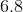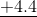# SSAT Middle Level Math : How to add fractions

## Example Questions

### Example Question #1331 : Ssat Middle Level Quantitative (Math)

Which of the following is the sum of sixty-nine tenths, sixty-nine thousandths, and sixty-nine ten-thousandths?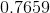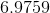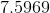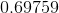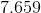Explanation:

Sixty-nine tenths, sixty-nine thousandths, and sixty-nine ten-thousandths can be rewritten as 6.9, 0.069, and 0.0069, respectively. Add, appending zeroes at the end of each number as needed: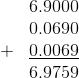### Example Question #3 : Additive Inverse Property

Which of the following statements demonstrates the inverse property of addition?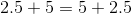None of the examples in the other responses demonstrates the inverse property of addition.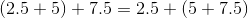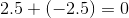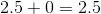Explanation:

The inverse property of addition states that for every real number, a number exists, called the additive inverse, such that the number and its inverse have sum 0. Of the statements given, onlydemonstrates this property, so it is the correct choice.

### Example Question #3 : Additive Identity Property

Which of the following statements demonstrates the identity property of addition?None of the examples in the other responses demonstrates the identity property of addition.Explanation:

The identity property of addition states that there is a number 0, called the additive identity, that can be added to any number to yield that number as the sum. Of the four statements,demonstrates this property.

### Example Question #1332 : Ssat Middle Level Quantitative (Math)

Which of the following is the sum of two-thirds and one-fifth?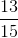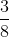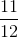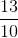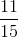Explanation:

Since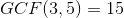, rewrite each fraction as its equivalent in fifteenths and add the numerators: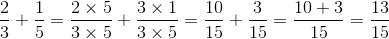### Example Question #178 : Decimals

Which of the following is the sum of nine tenths and nineteen thousandths?

The correct response is not given in the other choices.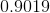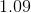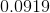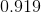Explanation:

Nine tenths is equal to 0.9; nineteen thousandths is equal to 0.019. Add them, rewriting 0.9 as 0.900: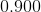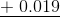### Example Question #22 : Problem Solving

The time is now 11:17 AM. What time will it be in three hours and twenty-four minutes?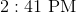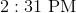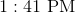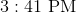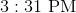Explanation:

The time is 11:17 AM. In 24 minutes, the minutes will read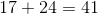, so it wil be 11:41 AM. Let's then add three hours to it.

The time will be 2:41 PM.

### Example Question #1 : How To Add Fractions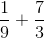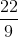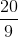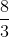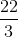Explanation:

Rewrite using the lowest comon denominator. Since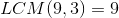: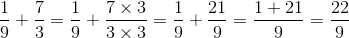### Example Question #6 : Addition With Fractions

Solve: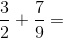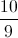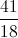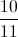Explanation:

The least common multiple of 2 and 9 is 18.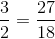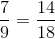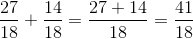### Example Question #2 : How To Add Fractions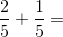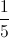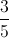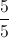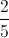Explanation:### Example Question #3 : How To Add Fractions

Which of the following expressions is equal to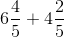?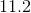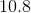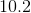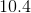Explanation:

Rewrite both numbers as decimals: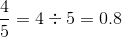, so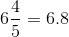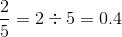, so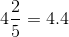Add the decimals vertically, lining up the decimal points: